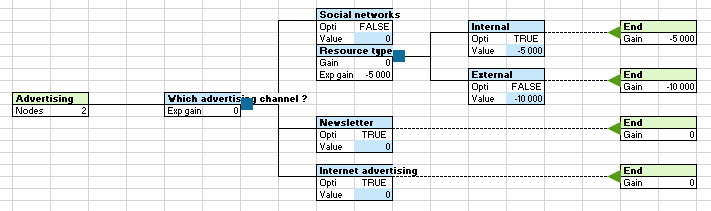# Decision trees

Use this tool to represent different possible solutions to a problem and their potential results into a graph and take the best decision. Available in Excel with the XLSTAT software.## What is a decision tree?

A decision tree is a diagram represented by a set of nodes interconnected through branches. It allows its creator to evaluate different possible actions according to their cost, benefit and probability. It usually begins with a node from which several possible outcomes arise. Each of these results leads to other nodes, children, from which other possibilities emanate. The resulting diagram recalls the shape of a tree.

## Options for Decision Trees in XLSTAT

Here is a brief description for each type of node, with their graphical representation, in block form, in XLSTAT:

### Different types of nodes

• Decision node: It is represented by a blue square. It illustrates a decision to be made among several possible choices (branches).
• Chance node: It is represented by a red circle. It offers different outcomes, each with a % of chance of occurrence. The % of chance must sum to 100.
• Logical node: It is represented by a yellow hexagone. It imposes a result or not according to the return of a logical formula.
• Reference node: It is represented by a purple rhombus. It refers to a subtree, that is, to another node with all of its children.
• End node: It is represented by a green triangle. It is the final result of a decision path. Here is an example of an end node.

### Computation modes

There are two calculation methods to help you in making a decision. You can choose to maximize your gain if you want to maximize your profit, or to minimize your gain if you want to optimize your cost. These two calculation methods are enhanced with the possibility of having a gain, the sum of costs and profits, but also a gain calculated from an exponential utility function.

In a risky universe, a perfectly rational individual makes investment decisions by maximizing the expectation (in the probabilistic sense of the term) of his utility function. This reflects the satisfaction generated by a given future wealth. In our case, we use the exponential utility function.

## Results for Decision Trees in XLSTAT

For each block of a tree, whether it is the block of the tree itself, a node block or a branch block, calculations are carried out for each modification. Their result is displayed directly in the form of formulas, in the blocks concerned. Unlike editable information in which the cell is colored, the information resulting from the calculation of a formula is on a white background. It is possible to replace formulas deleted by mistake by generating the tree again. All you have to do is open the tree parameterization dialog box and click on the OK button.

### Information available for each node

Each node includes different information that is available to you. You can choose to display all of this information or not. This setting will be discussed in the Building a tree section. In the meantime, here is a description of these different pieces of information. The concepts of calculation mode and utility function will be presented next.

• Cost: The cost of a node is the sum of all the costs of its parent nodes.
• Profit: The profit of a node is the sum of all the profits of its parent nodes.
• Gain: The gain of a node is the sum of its cost and its profit. It is therefore calculated according to the costs and profits of its parent nodes. The cost is a negative value and the profit a positive value.
• Expected gain: The expected gain of a node depends on the gain of its child nodes. It helps to make a decision according to the chosen calculation method.
• Gain with utility: The gain with utility of a node is calculated using the exponential utility function applied to the gain of this same node.
• Expected gain with utility: The expected gain with utility of a node depends on the gain with utility of its child nodes. It helps to make a decision according to the chosen calculation method.
• Referent leaf: Only in the case of a reference node, it is the name of the leaf where the referent node is located. If this sheet is in a different workbook than the one where the current tree is located, then the name of this workbook is also filled in.
• Referent tree: Only in the case of a reference node, it is the name of the tree containing the referent node.
• Referent node: Only in the case of a reference node, it is the name of the referring node.

### Optimal path

This tool is not yet available on the Mac platform.

The optimal path represents the path that best meets the chosen calculation mode. It depends on the expected gain, with utility or not, from each node. The choice of an optimal path for the expected gain or the expected gain with utility is done via the tree parameterization dialog box.

The optimal path, once activated, is highlighted on the tree. It can concern the entire tree or it can only start from a node that you have chosen by right-clicking. As long as the optimal tree is activated, it is automatically recalculated and its display updated, each time the tree is modified.### analice sus datos con xlstat

prueba gratuita de 14 días

Incluido en